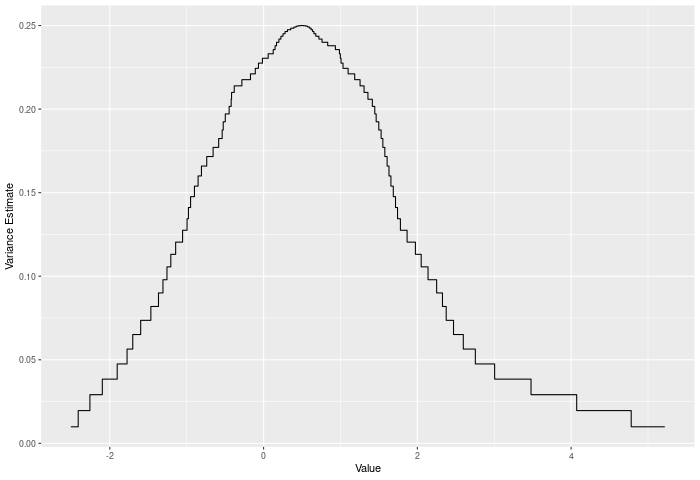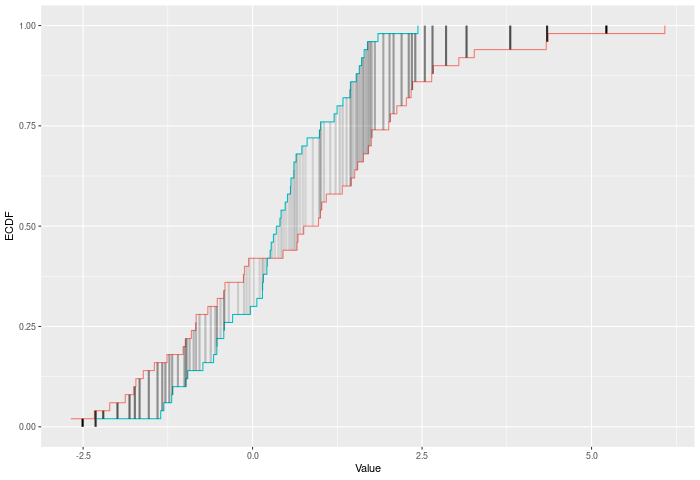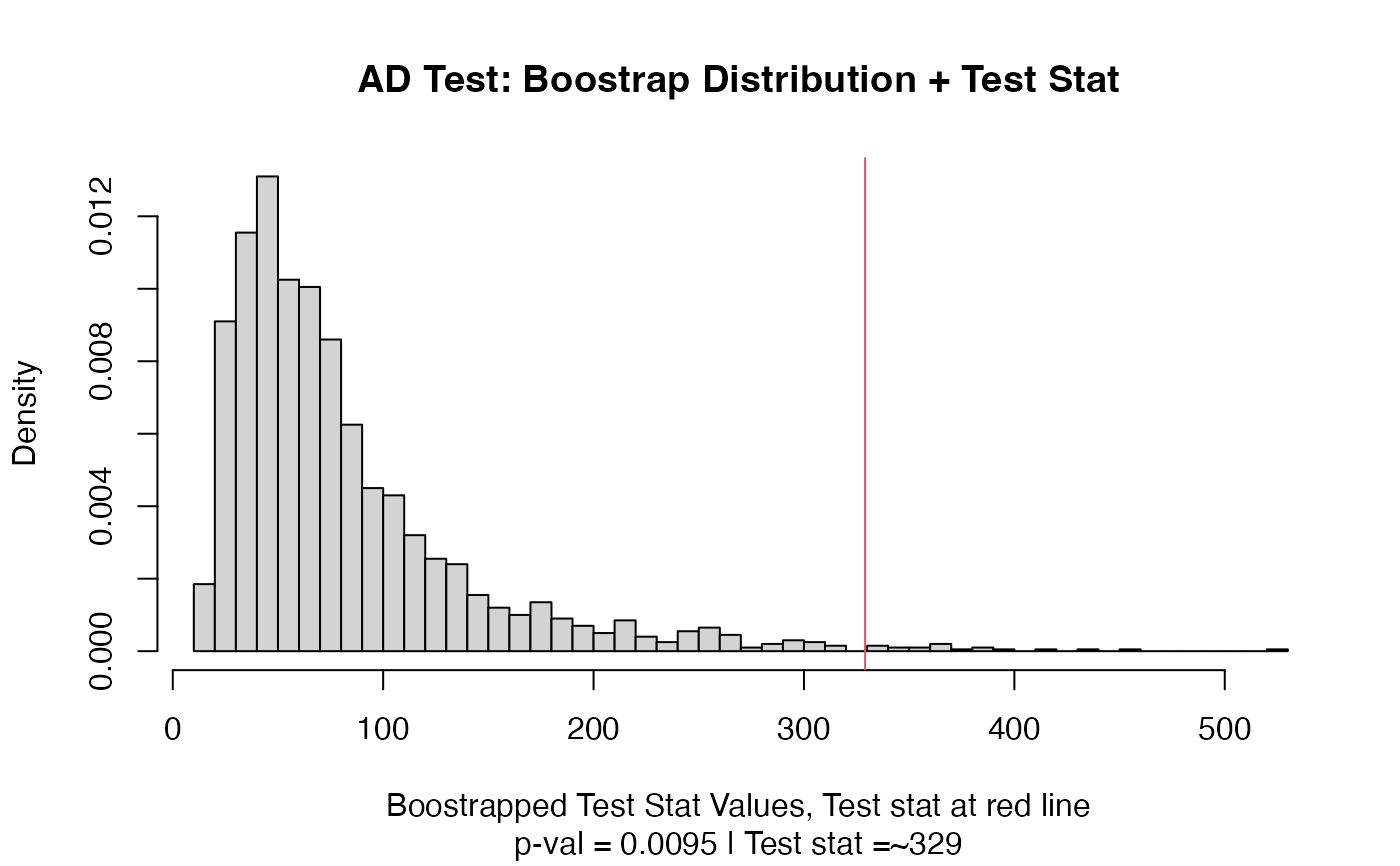A two-sample test based on the Anderson-Darling test statistic (ad_stat).

## Usage

ad_test(a, b, nboots = 2000, p = default.p, keep.boots = T, keep.samples = F)

ad_stat(a, b, power = def_power)

## Arguments

a

a vector of numbers (or factors -- see details)

b

a vector of numbers

nboots

Number of bootstrap iterations

p

power to raise test stat to

keep.boots

Should the bootstrap values be saved in the output?

keep.samples

Should the samples be saved in the output?

power

power to raise test stat to

## Value

Output is a length 2 Vector with test stat and p-value in that order. That vector has 3 attributes -- the sample sizes of each sample, and the number of bootstraps performed for the pvalue.

## Details

The AD test compares two ECDFs by looking at the weighted sum of the squared differences between them -- evaluated at each point in the joint sample. The weights are determined by the variance of the joint ECDF at that point, which peaks in the middle of the joint distribution (see figure below). Formally -- if E is the ECDF of sample 1, F is the ECDF of sample 2, and G is the ECDF of the joint sample then $$AD = \sum_{x \in k} \left({|E(x)-F(x)| \over \sqrt{2G(x)(1-G(x))/n} }\right)^p$$ where k is the joint sample. The test p-value is calculated by randomly resampling two samples of the same size using the combined sample. Intuitively the AD test improves on the CVM test by giving lower weight to noisy observations.

In the example plot below, we see the variance of the joint ECDF over the range of the data. It clearly peaks in the middle of the joint sample.In the example plot below, the AD statistic is the weighted sum of the heights of the vertical lines, where weights are represented by the shading of the lines.Inputs a and b can also be vectors of ordered (or unordered) factors, so long as both have the same levels and orderings. When possible, ordering factors will substantially increase power.

## Functions

• ad_test: Permutation based two sample Anderson-Darling test

• ad_stat: Permutation based two sample Anderson-Darling test

dts_test() for a more powerful test statistic. See cvm_test() for the predecessor to this test statistic. See dts_test() for the natural successor to this test statistic.

## Examples

set.seed(314159)
vec1 = rnorm(20)
vec2 = rnorm(20,0.5)
out
#> Test Stat   P-Value
#>  329.0609    0.0095
summary(out)
#> =========================
#> Test Statistic: 329.0609
#>        P-Value: 0.0095 *
#> - - - - - - - - - - - - -
#>      n1      n2 n.boots
#>      20      20    2000
#> =========================
#> Test stat rejection threshold for alpha = 0.05 is: 210.8735
#> Null rejected: samples are from different distributions
plot(out)# Example using ordered factors
vec1 = factor(LETTERS[1:5],levels = LETTERS,ordered = TRUE)
vec2 = factor(LETTERS[c(1,2,2,2,4)],levels = LETTERS, ordered=TRUE)